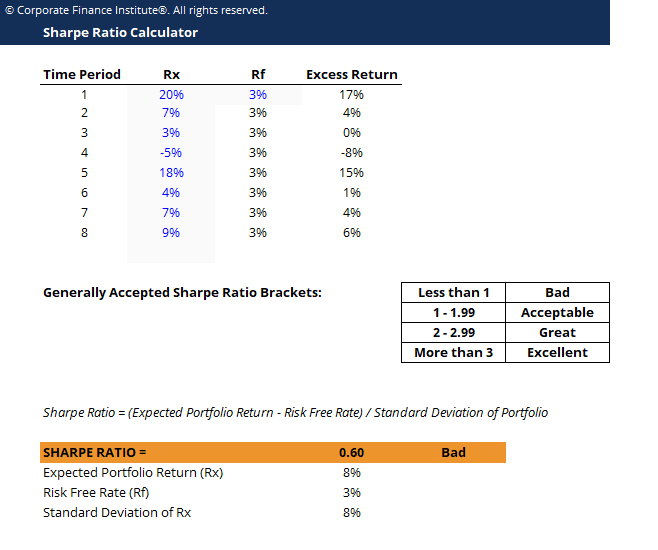# Sharpe Ratio Calculator Template

## Sharpe Ratio Calculator Template

This sharpe ratio calculator template demonstrates the calculation of sharpe ratio to determine an investment’s performance relative to risk.

Below is a preview of the sharpe ratio calculator template:### Download the Free Template

Enter your name and email in the form below and download the free template now!

### Sharpe Ratio Calculator

Download the free Excel template now to advance your finance knowledge!

The Sharpe ratio is commonly used as a means of calculating the performance of an investment after adjusting for its risk. This allows investments of different risk profiles to be compared against each other.

In the Sharpe Ratio, a higher value means greater returns for the portfolio relative to the inherent risk. This also means a better investment. Because of the simplicity of the formula, the Sharpe Ratio can be used to evaluated a single stock, or an entirely diversified portfolio.

### Sharpe Ratio formula

Sharpe Ratio = (Rx – Rf) / StdDev Rx

Where:

• Rx = Expected portfolio return
• Rf = Risk free rate of return
• StdDev Rx = Standard deviation of portfolio return / volatility

### More Free Templates

For more resources, check out our business templates library to download numerous free Excel modeling, PowerPoint presentation and Word document templates.

• Excel Modeling Templates
• PowerPoint Presentation Templates
• Transaction Document Templates

### Financial Analyst Certification

Become a certified Financial Modeling and Valuation Analyst (FMVA)® by completing CFI’s online financial modeling classes and training program!